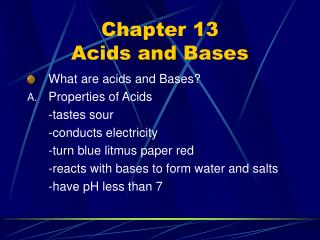# Chapter 13 Acids and Bases - PowerPoint PPT PresentationDownload PresentationChapter 13 Acids and Bases

Chapter 13 Acids and BasesDownload Presentation## Chapter 13 Acids and Bases

- - - - - - - - - - - - - - - - - - - - - - - - - - - E N D - - - - - - - - - - - - - - - - - - - - - - - - - - -
##### Presentation Transcript

1. Chapter 13Acids and Bases • What are acids and Bases? • Properties of Acids -tastes sour -conducts electricity -turn blue litmus paper red -reacts with bases to form water and salts -have pH less than 7

2. Naming Acids • Two Types of Acids a. Binary Acid -contains only H and one other element Naming Binary Acids 1. Name begins with prefix hydro- 2. Root of second element follows hydro and ends with the suffix -ic

3. Examples of Binary Acids • Hydrofluoric acid HF • Hydrochloric acid HCl • Hydrobromic acid HBr • Hydrosulfuric acid H2S

4. Acids cont. b. Oxyacid - acid that contains H, O, and a third element,usually a nonmetal Naming Oxyacids 1. Root of the anion plus the suffix –ic followed by the word acid

5. Examples of Oxyacids • Acetic acid acetate • Carbonic acid carbonate • Nitric acid nitrate • Phosphoric acid phosphate • Sulfuric acid sulfate

6. Properties of Bases • tastes bitter • Feels slippery • Turns red litmus paper blue • Reacts with acids to form water and salts • Have a pH greater than 7

7. Bases cont. • Naming Bases - Element name followed by hydroxide - There are a few exceptions: Ammonia NH3 Aniline C6H5NH2

8. Acid-Base Theories • Arrhenius Acids and Bases 1. Arrhenius Acid acids in water produce H+ ions HNO3(aq) H+(aq) + NO3- 2. Arrhenius Base bases in water produce OH- KOH(s) K+(aq) + OH-(aq)

9. Cont. • Bronsted-Lowry Acids and Bases -Acids are proton(H+) donors H2CO3(aq)+H2O(l)H3O+(aq)+HCO3-(aq) (acid) (base) (C.A.) (C.B.) -Bases are proton acceptor NH3(aq) + H2O(l)  NH4+(aq) + OH-(aq) base acid C.A. C.B. Conjugate acid- ion formed when a base has accepted a H+ Conjugate base- ion formed when an acid has donated a H+

10. How are weak acids and bases compared? • Acid-dissociation constant(Ka) • ratio of concentrations of the products to the reactants for a weak acid • Example CH3COOH(aq) + H2O(l) CH3COO-(aq) + H3O+(aq) Ka= [CH3COO-] [H3O+] [CH3COOH]

11. Cont. • Base-dissociation constant(Kb) -ratio of concentrations of the products to the reactants for a weak base NH3(aq) +H2O(l) NH4+(aq) + OH-(aq) Kb= [NH4+] [OH-] [NH3]

12. The pH scale • Is the negative of the common logarithm of the hydronium ion concentration • Ranges from 0-14 • We use the following equation: pH= -log[H3O+]

13. Self-Ionization Constant of Water 2H2O(l)  H3O+(aq) + OH-(aq) Kw= [H3O+] [OH-] Kw = 1.00 x 10-14 at 25° C

14. Determining [H3O+] and [OH-] with Kw • Example What is [OH-] in a 3.00x 10-5 M solution of HCl? HCl(g) + H2O(l)  H3O+(aq) +Cl-(aq) 2H20(l)  H3O+(aq) + OH-(aq) What do we know? Kw= 1.00 x 10-14 [H3O+]= 3.00 x 10-5

15. Using Logarithms in pH calculations • 1. Calculating pH from [H3O+] pH= -log [H3O+] 2. Calculating [H3O+] from pH [H3O+] = 10-pH

16. Examples of pH • What is the pH of a .00010 M solution of HNO3, a strong acid? • [HNO3]= .00010 M or 1.0 x 10-4 M pH= -log[1.0 x10-4] pH= 4.00

17. Examples cont. What is the pH of a .0136 M solution of KOH, a strong base? [OH-]= .0136 M Kw= 1.00 x 10-14 Kw= [H3O+][OH-] pOH= -log[OH-] pH + pOH = 14

18. Calculating [H3O+] and [OH-] from pH What are the concentrations of the hydronium and hydroxide ions in a sample of rain that has a pH of 5.05? [H3O+] = 10-5.05 = 8.9 x 10-6 M Since pH + pOH = 14 pOH= 8.95 so [OH-] = 10-8.95 = 1.1 x 10-9 M

19. Calculating Ka/Kb for a weak acid or base A vinegar sample is found to have .837 M CH3COOH. Its hydronium concentration is found to be 3.86 x 10-3 mol/L. Calculate Ka for acetic acid. What do we know? [CH3COOH]= .837 M [H3O+]= 3.86 x 10-3 M CH3COOH(aq)+ H2O(l)H3O+(aq)+CH3COO-(aq)

20. Neutralization and Titrations • Neutralization Reaction • a reaction between an acid and a hydroxide base that results in a salt and water HCl + NaOH  NaCl + H2O

21. Titrations Titrations • a procedure in which a solution of known concentration is used to determine the concentration of a second unknown solution Equivalence Point - the point at which a neutralization reaction is complete

22. Titrations cont. • How do we know when the equivalence point has been reached? • You use an indicator • A substance that changes its color as it reacts with either an acid or base • The instant the indicator changes color is called the End Point • Common indicators are red cabbage juice, litmus, bromthymol blue, and phenolphthalein

23. Titration Calculations • Suppose that in the titration of 40. mL of vinegar, 20.mL of .50 M NaOH were needed to reach the equivalence point. What is the molarity of the acetic acid in the vinegar? NaOH(aq)+CH3COOH(aq)NaCH3COO(aq) + H2O(l) What do we know? Volume of NaOH= 20. Ml [NaOH]= .50 M Volume of vinegar = 40. ml